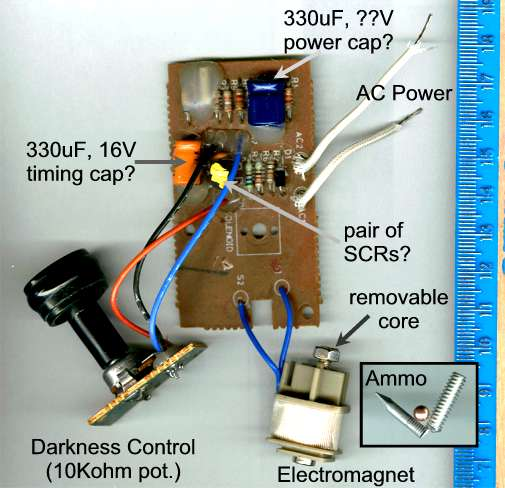# A Coilgun Made From A Toaster!

Replaced a toaster a while back. Took the old one apart to see if there were any interesting parts in it. Turns out the modern toaster is a surprisingly sophisticated hunk of electronics.

Indeed, this lowly toaster contained a complete coilgun (aka gauss rifle, rail gun, asynchronous linear induction motor ... )! Power supply, "high voltage" supply, coil everything.

I was expecting a switch, heater coil and a bi-metal temperature sensor that releases the spring loaded toast tray when it got hot enough.

Instead, there was a switch (activated when the bread is loaded) suppling power to the heater coil and the circuit board below.The circuit appears to contain two basic functions.

### A Timer Circuit

• Sets the time for cookin' your toast via the 10K potentiometer and the orange 330uF, 16V capacitor.
• Minimum duration is about 40 seconds, the maximum about 2.5 minutes.

### A "High Power" Supply and Coil

• A 330uF capacitor (dark blue, upper right corner of picture) that is charged to 17.7 volts.
• The capacitor is dumped via the SCR(s?) through the electromagnet's coil when the timer times out.
• The electromagnet releases a catch on the toast tray which ejects the toast and shuts of power to the circuit.

The hole thing is powered by the 120V AC main. Since I've removed the main power switch the board cycles continously as long as power is connected.

The electromagnet's iron core is a screw that attached the coil to the circuit board. The screw was removed to convert the iron-cored electromagnet to a launch coil. The ID of the resulting hole (and the OD of the screw) is 0.235". The coil has a resistance of 166 Ohms and is about 1/2 inch in diameter. If the coil wire is 36G with 0.423Ohm resistance per foot then the wire is about 390 feet long and makes about 3000 turns. If the coil wire is 40G with 1.070Ohm resistance per foot then the wire is about 155 feet long and makes about 1200 turns.

For ammo, I used BBs (which are too small), a hunk of nail and a hunk of bolt. A ~1/2 inch length of 1/4 inch copper tube was hot glued to one side of the coil to provide a loading platform (breech) for the ammo.

To measure the muzzle velocity I fired the gun horizontally from a height (h) of 3 feet and measured the horizontal distance (x) where the projectile hit the ground. From this the muzzle (horizontal) velocity can be calculated with;

 vmuzzle = x = x = 4x (x & h in feet) SQRT(2h/g) SQRT(h/16) SQRT(h)

Of course, once you know the velocity you can calculate the kinetic energy (KE) of the projectile with;

 KE = 1/2mv2 where m=mass and v=velocity

And, the potential energy (PE) stored in the capacitor is;

 PE = 1/2CV2     where C=capacitance in Farads and V=voltage = 1/2(330x10-6)(17.7)2 = 0.052 Joules

Results for my three projectiles;

Ammo OD
(inch)
Length
(inch)
Mass
(g)
Horizontal
Distance While
Falling 3' (ft)
Muzzle
Velocity
(ft/sec)
Muzzle
Velocity
(m/sec)
Kinetic Energy
(kgm2/s2)
Efficiency
BB 0.177 0.177 0.37 2.0 4.6 1.4 0.00036 0.7%
nail 0.12 0.69 ~1 5.0 11.5 3.5 0.0061 12%
bolt 0.18 0.62 ~2 5.5 12.7 3.9 0.015 29%

The 12.7fps muzzle velocity with the hunk of bolt is about the same as dropping the bolt from a height of 2.5 feet. (Pretty craptacular eh?)

Hmmmm, if I attach this coil to the photoflash capacitor from a disposable camera, a 120uF capacitor at ~330V, then the muzzle velocity for the bolt should be;

 (12.7fps) SQRT[(330V)2(120uF)] / SQRT[(17.7V)2(330uF)] = 143fps

I wonder if the coil will handle 6.5J of energy?

Overall, not bad for a toaster. A cheap BB gun fires at ~300 ft/sec but it won't cook you a bagel in the morning.